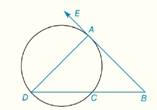Chapter 6.CR, Problem 7CRElementary Geometry For College St...

7th Edition
Alexander + 2 others
ISBN: 9781337614085

Solutions

Chapter
SectionElementary Geometry For College St...

7th Edition
Alexander + 2 others
ISBN: 9781337614085
Textbook Problem

In Review Exercises 5 to 10, B A → is tangent to the circle at point A in the figure shown. m ∠ E A D = 70 ° ,   m ∠ B = 30 ° ,     m A C ^ = ?To determine

To find: mAC^

Explanation

Theorem:

1) The measure of an angle formed when two secants intersect at a point outside the circle is one-half the difference of the measures of two intercepted arcs.

2) The measure of an inscribed angle of a circle is one-half the measure of its intercepted arc.

Calculation:

Given that

mEAD=70°, mB=30° and BA is tangent to the circle at point A.

Subtract 70° from both the sides

Still sussing out bartleby?

Check out a sample textbook solution.

See a sample solution

The Solution to Your Study Problems

Bartleby provides explanations to thousands of textbook problems written by our experts, many with advanced degrees!

Get Started

In Problems 35-42, simplify each complex fraction. 41.

Mathematical Applications for the Management, Life, and Social Sciences

Find all degree solutions. 2cos22cos21=0

Trigonometry (MindTap Course List)

For f(x)=xx+1,f(x)=. a) 1 b) x2(x+1)2 c) 1(x+1)2 d) 1(x+1)2

Study Guide for Stewart's Single Variable Calculus: Early Transcendentals, 8th